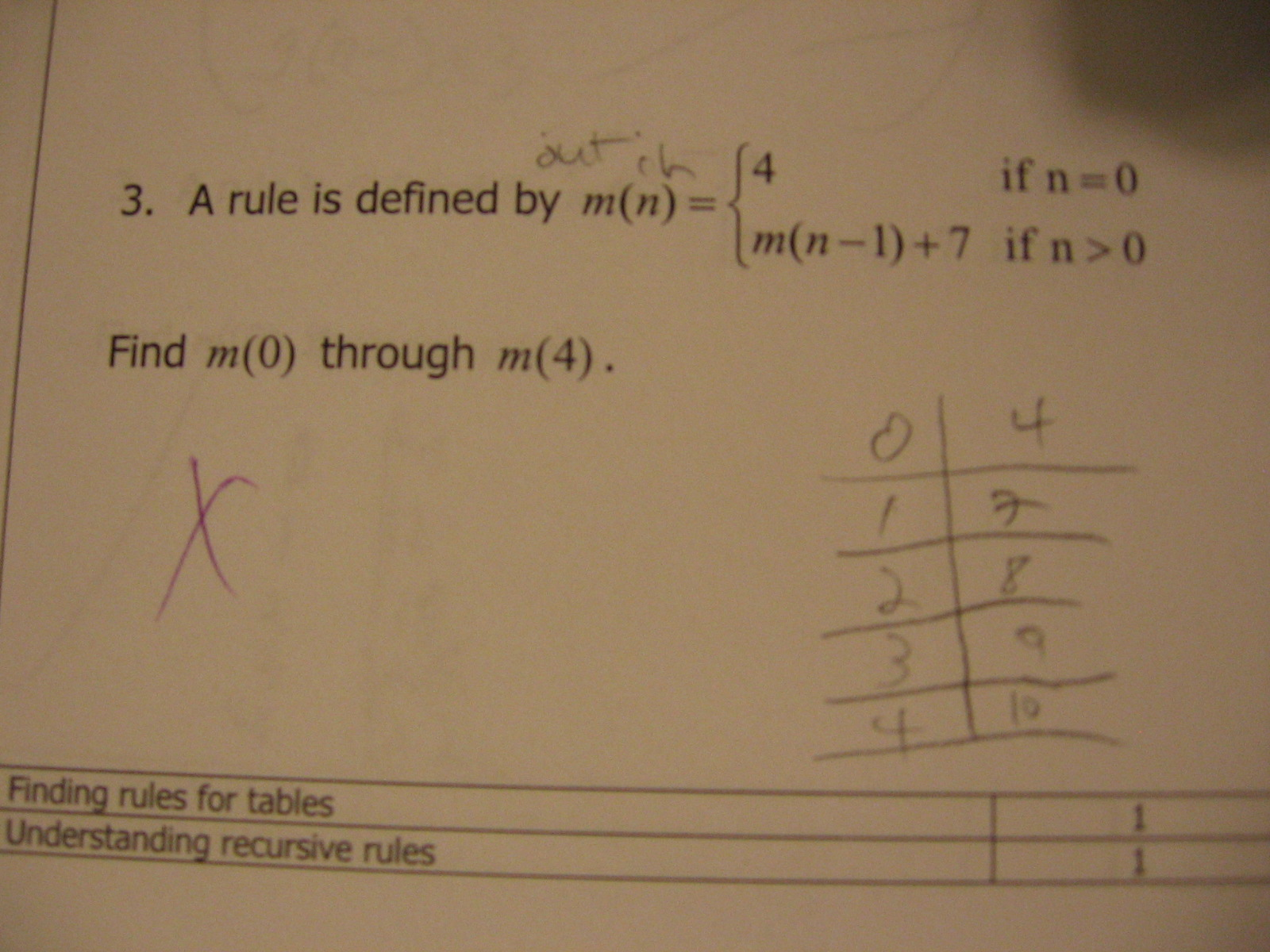Categories

# Following a recursively defined ruleWhat does the student understand about recursive functions? What’s she confused by? How would you help?

## 6 replies on “Following a recursively defined rule”

At first I thought the student was treating this as m times n-1, so when n=1 that part is zero. But instead it looks like they’re just ignoring the m altogether (sometimes teachers say this – “it’s just a name”), n=3 gives 3-1+7=9. Can’t tell what the stu thinks about recursion. I’d be interested to see what they would do with a now-next kind of rule.
First term = 4. Next = Previous + 7.

I’d agree that the student is doing the time honored “if it doesn’t make sense, work around it” approach, which is sort of consistent with the idea that “m(n)” doesn’t mean multiply m by n *all* the time. How *would* you explain this?David Pattersonsays:

I think a different spacing/arranging might help.

if n=0, m(n) = 4
if n>0, m(n) = m(n-1)+7

I think m(n-1)+7 is being read as m = (n-1)+7

I realize that students should be able to read the problem the way it was written. Was this the first time the student saw it in that format/spacing?tieandjeanssays:

This page looks similar to the last recursive rule question, which is phrased similarly, so I’m going to assume some familiarity with the notation.
David – Your phrasing stands a good chance of improving this error, but I would wince before putting an = sign next to the function expression. My gut feeling is that using an = sign obscures the idea that functions EVALUATE to something based on the value of n, which is subtly different than a solvable “y = ”

You can dismiss this as non-mathy, but I’d like some langauge in there.

m(n) = { if n = 0: 4
else: m(n-1) }

Typing that out, I’ve also decided that I like the conditions on the left and the value on the right. Is that backwards from how we normally write peicewise functions?

That notation is problematic, I think, because it implies that the domain includes numbers that are less than 0.tieandjeanssays:

m(n) = { if n = 0: 4
if n > 0 : m(n-1)
}

Does that change address the domain issue? You could also just add “for n in Natural” or V n s.t. n e Z && n > 0, or any other statement of set membership.